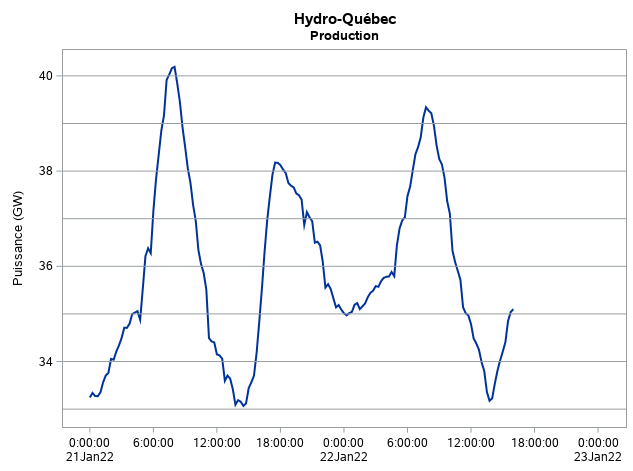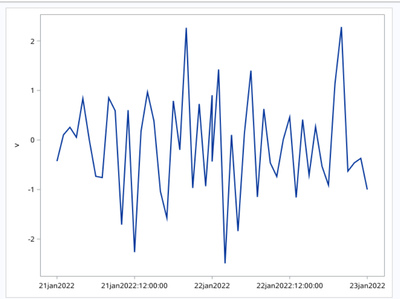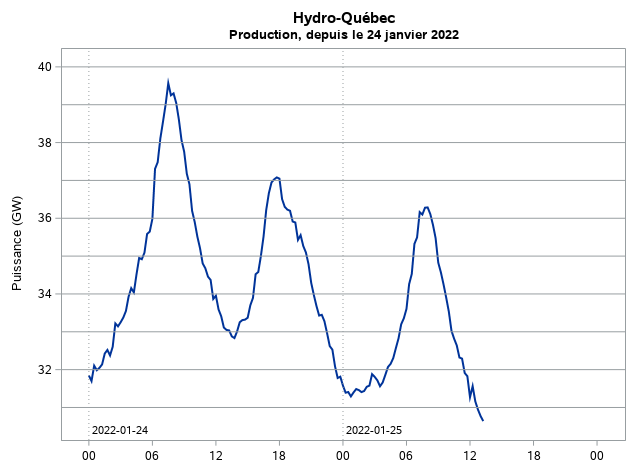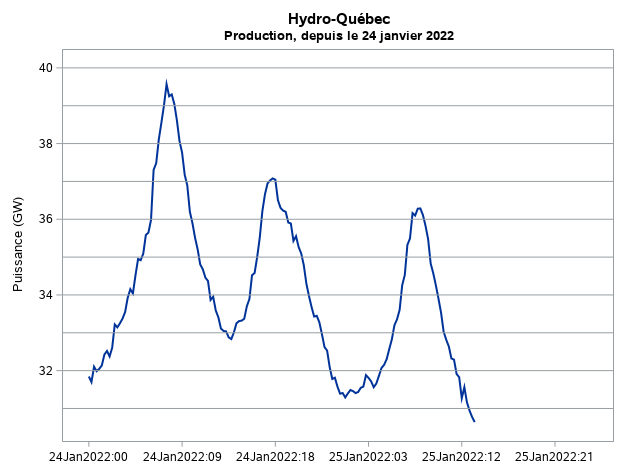PGStats
Opal | Level 21

## Adjusting the auto format of tick values on times axes in SGPLOT

Hi, I have the following code

``````title "Hydro-Québec";
title2 "Production";
proc sgplot data=demande;
series x=date y=demandeGW / lineattrs=(thickness=2);
xaxis type=time display=(nolabel) offsetmin=0.05 offsetmax=0.05;
yaxis offsetmin=0.05 offsetmax=0.05;
refline 33 to 40 by 1 / axis=y noclip;
run;
``````

Variable date has DATETIME. format. I like the resulting output:But I would like to get rid of the ":00:00" on the X axis, but to keep the date values on a separate line.

Any ideas?

PG
6 REPLIES 6

## Re: Adjusting the auto format of tick values on times axes in SGPLOT

What about a user-defined PICTURE format in PROC FORMAT? Selecting the DATETIME PICTURE option with the date and time components should get you what you want.PGStats
Opal | Level 21

## Re: Adjusting the auto format of tick values on times axes in SGPLOT

Thanks @SASKiwi . Good idea, but it seems like user-defined PICTURE formats are not expanded by proc SGPLOT on TIME axes. I also tried option INTERVAL=HOUR in the hope that it would reduce the displayed resolution of time values - it didn't.

``````proc format;
picture myTime (default=12)
low-high = '%d%b%y %H' (datatype=datetime);
run;

title "Hydro-Québec";
title2 "Production";
proc sgplot data=demande;
series x=date y=demandeGW / lineattrs=(thickness=2);
xaxis type=time display=(nolabel) offsetmin=0.05 offsetmax=0.05 valuesformat=myTime.;
yaxis offsetmin=0.05 offsetmax=0.05;
refline 33 to 40 by 1 / axis=y noclip;
run;``````PG

## Re: Adjusting the auto format of tick values on times axes in SGPLOT

What about GPLOT? Same problem? Have you checked your format with PROC PRINT or similar to confirm it has defined correctly?Ksharp
Super User

## Re: Adjusting the auto format of tick values on times axes in SGPLOT

Hi PGStats , Like this ?

``````

data have;
do date='21jan2022'd to '22jan2022'd ;
do time='00:00:00't to '24:00:00't by '01:00:00't;
datetime=dhms(date,0,0,time);
v=rand('normal');output;
end;
end;
format datetime datetime.;
run;

proc sgplot data=have;
series x=datetime y=v / lineattrs=(thickness=2);
xaxis type=linear display=(nolabel) offsetmin=0.05 offsetmax=0.05
values=('21jan2022:00:00:00'dt to '22jan2022:24:00:00'dt by '12:00:00't)
valuesdisplay=('21jan2022' '21jan2022:12:00:00' '22jan2022'  '22jan2022:12:00:00' '23jan2022')   ;
yaxis offsetmin=0.05 offsetmax=0.05;
run;``````DanH_sas
SAS Super FREQ

## Re: Adjusting the auto format of tick values on times axes in SGPLOT

What do you get if you set INTERVAL=HOUR on the XAXIS statement?PGStats
Opal | Level 21

## Re: Adjusting the auto format of tick values on times axes in SGPLOT

Thanks to everyone. What I ended up doing:

``````/* Create labels based on data */
proc sql noprint;
select
put (datepart(min(date)), nldate.),
put (datepart(min(date)), yymmdd10.),
intnx ("dtday", min(date), 0, "B") format=15.,
put (datepart(min(date)) + 1, yymmdd10.),
intnx ("dtday", min(date), 1, "B") format=15.
into
:titledate trimmed,
:datelabel1 trimmed,
:dateref1 trimmed,
:datelabel2 trimmed,
:dateref2 trimmed
from demande;
quit;

title "Hydro-Québec";
title2 "Production, depuis le &titledate.";
proc sgplot data=demande;
series x=date y=demandeGW / lineattrs=(thickness=2);
xaxis type=time display=(nolabel) offsetmin=0.05 offsetmax=0.05 valuesformat=tod2. ;
yaxis offsetmin=0.05 offsetmax=0.05;
refline &dateref1. &dateref2. /
axis=x lineattrs=(pattern=dot) labelloc=inside labelpos=min
label=("&datelabel1." "&datelabel2.") labelattrs=(size=8);
refline 20 to 40 by 1 / axis=y;
run;``````I won't mark my own answer as a solution, since it is close but not quite what I wanted, i.e. automatic tick labels on two lines outside the data frame without the ":00:00".

Btw the INTERVAL=HOUR option created full date:hour tick labels on a single line.PG
Discussion stats
• 6 replies
• 649 views
• 5 likes
• 4 in conversation